## Introduction

`qgcomp` is a package to implement g-computation for analyzing the effects of exposure mixtures. Quantile g-computation yields estimates of the effect of increasing all exposures by one quantile, simultaneously. This, it estimates a “mixture effect” useful in the study of exposure mixtures such as air pollution, diet, and water contamination.

Using terminology from methods developed for causal effect estimation, quantile g-computation estimates the parameters of a marginal structural model that characterizes the change in the expected potential outcome given a joint intervention on all exposures, possibly conditional on confounders. Under the assumptions of exchangeability, causal consistency, positivity, no interference, and correct model specification, this model yields a causal effect for an intervention on the mixture as a whole. While these assumptions may not be met exactly, they provide a useful roadmap for how to interpret the results of a qgcomp fit, and where efforts should be spent in terms of ensuring accurate model specification and selection of exposures that are sufficient to control co-pollutant confounding.

## How to use the `qgcomp` package

Here we use a running example from the `metals` dataset from the from the package `qgcomp` to demonstrate some features of the package and method.

Namely, the examples below demonstrate use of the package for: 1. Fast estimation of exposure effects under a linear model for quantized exposures for continuous (normal) outcomes 2. Estimating conditional and marginal odds/risk ratios of a mixture effect for binary outcomes 3. Adjusting for non-exposure covariates when estimating effects of the mixture 4. Allowing non-linear and non-homogenous effects of individual exposures and the mixture as a whole by including product terms 5. Using qgcomp to fit a time-to-event model to estimate conditional and marginal hazard ratios for the exposure mixture

For analogous approaches to estimating exposure mixture effects, illustrative examples can be seen in the `gQWS` package help files, which implements weighted quantile sum (WQS) regression, and at https://jenfb.github.io/bkmr/overview.html, which describes Bayesian kernel machine regression.

The `metals` dataset from the from the package `qgcomp`, comprises a set of simulated well water exposures and two health outcomes (one continuous, one binary/time-to-event). The exposures are transformed to have mean = 0.0, standard deviation = 1.0. The data are used throughout to demonstrate usage and features of the `qgcomp` package.

``````library("qgcomp")
library("knitr")
library("ggplot2")
data("metals", package="qgcomp")
``````
``````##       arsenic      barium    cadmium    calcium    chloride    chromium
## 1  0.09100165  0.08166362 15.0738845 -0.7746662 -0.15408335 -0.05589104
## 2  0.17018302 -0.03598828 -0.7126486 -0.6857886 -0.19605499 -0.03268488
## 3  0.13336869  0.09934014  0.6441992 -0.1525231 -0.17511844 -0.01161098
## 4 -0.52570747 -0.76616263 -0.8610256  1.4472733  0.02552401 -0.05173287
## 5  0.43420529  0.40629920  0.0570890  0.4103682 -0.24187403 -0.08931824
## 6  0.71832662  0.19559582 -0.6823437 -0.8931696 -0.03919936 -0.07389407
##        copper       iron         lead  magnesium   manganese     mercury
## 1  1.99438050 19.1153352 21.072630908 -0.5109546  2.07630966 -1.20826726
## 2 -0.02490169 -0.2039425 -0.010378362 -0.1030542 -0.36095395 -0.68729723
## 3  0.25700811 -0.1964581 -0.063375935  0.9166969 -0.31075240  0.44852503
## 4  0.75477075 -0.2317787 -0.002847991  2.5482987 -0.23350205  0.20428158
## 5 -0.09919923 -0.1698619 -0.035276281 -0.5109546  0.08825996  1.19283834
## 6 -0.05622285 -0.2129300 -0.118460981 -1.0059145 -0.30219838  0.02875033
##      nitrate    nitrite         ph    selenium     silver      sodium
## 1  1.3649492 -1.0500539 -0.7125482  0.23467592 -0.8648653 -0.41840695
## 2 -0.1478382  0.4645119  0.9443009  0.65827253 -0.8019173 -0.09112969
## 3 -0.3001660 -1.4969868  0.4924330  0.07205576 -0.3600140 -0.11828963
## 4  0.3431814 -0.6992263 -0.4113029  0.23810705  1.3595205 -0.11828963
## 5  0.0431269 -0.5041390  0.3418103 -0.02359910 -1.6078044 -0.40075299
## 6 -0.3986575  0.1166249  1.2455462 -0.61186017  1.3769466  1.83722597
##      sulfate total_alkalinity total_hardness       zinc mage35          y
## 1 -0.1757544      -1.31353389    -0.85822417  1.0186058      1 -0.6007989
## 2 -0.1161359      -0.12699789    -0.67749970 -0.1509129      0 -0.2022296
## 3 -0.1616806       0.42671890     0.07928399 -0.1542524      0 -1.2164116
## 4  0.8272415       0.99173604     1.99948142  0.1843372      0  0.1826311
## 5 -0.1726845      -0.04789549     0.30518957 -0.1529379      0  1.1760472
## 6 -0.1385631       1.98616621    -1.07283447 -0.1290391      0 -0.4100912
##   disease_time disease_state
## 1 6.168764e-07             1
## 2 4.000000e+00             0
## 3 4.000000e+00             0
## 4 4.000000e+00             0
## 5 1.813458e+00             1
## 6 2.373849e+00             1
``````

### Example 1: linear model

``````# we save the names of the mixture variables in the variable "Xnm"
Xnm <- c(
'sodium','zinc'
)
covars = c('nitrate','nitrite','sulfate','ph', 'total_alkalinity','total_hardness')

# Example 1: linear model
# Run the model and save the results "qc.fit"
system.time(qc.fit <- qgcomp.noboot(y~.,dat=metals[,c(Xnm, 'y')], family=gaussian()))
``````
``````## Including all model terms as exposures of interest
``````
``````##    user  system elapsed
##   0.023   0.001   0.030
``````
``````#   user  system elapsed
#  0.011   0.002   0.018

# contrasting other methods with computational speed
# WQS regression
#system.time(wqs.fit <- gwqs(y~NULL,mix_name=Xnm, data=metals[,c(Xnm, 'y')], family="gaussian"))
#   user  system elapsed
# 35.775   0.124  36.114

# Bayesian kernel machine regression (note that the number of iterations here would
#  need to be >5,000, at minimum, so this underestimates the run time by a factor
#  of 50+
#system.time(bkmr.fit <- kmbayes(y=metals\$y, Z=metals[,Xnm], family="gaussian", iter=100))
#   user  system elapsed
# 81.644   4.194  86.520

#first note that qgcomp is very fast

# View results: scaled coefficients/weights and statistical inference about
# mixture effect
qc.fit
``````
``````## Scaled effect size (positive direction, sum of positive coefficients = 0.39)
##  calcium     iron   barium   silver  arsenic  mercury   sodium chromium
##  0.72216  0.06187  0.05947  0.03508  0.03447  0.02451  0.02162  0.02057
##  0.01328  0.00696
##
## Scaled effect size (negative direction, sum of negative coefficients = -0.124)
## magnesium    copper      lead manganese  selenium
##  0.475999  0.385299  0.074019  0.063828  0.000857
##
## Mixture slope parameters (Delta method CI):
##
##              Estimate Std. Error Lower CI Upper CI t value  Pr(>|t|)
## (Intercept) -0.356670   0.107878 -0.56811 -0.14523 -3.3062 0.0010238
## psi1         0.266394   0.071025  0.12719  0.40560  3.7507 0.0002001
``````

One advantage of quantile g-computation over other methods that estimate “mixture effects” (the effect of changing all exposures at once), is that it is very computationally efficient. Contrasting methods such as WQS (`gWQS` package) and Bayesian Kernel Machine regression (`bkmr` package), quantile g-computation can provide results many orders of magnitude faster. For example, the example above ran 3000X faster for quantile g-computation versus WQS regression, and we estimate the speedup would be several hundred thousand times versus Bayesian kernel machine regression.

Quantile g-computation yields fixed weights in the estimation procedure, similar to WQS regression. However, note that the weights from `qgcomp.noboot` can be negative or positive. When all effects are linear and in the same direction (“directional homogeneity”), quantile g-computation is equivalent to weighted quantile sum regression in large samples.

The overall mixture effect from quantile g-computation (psi1) is interpreted as the effect on the outcome of increasing every exposure by one quantile, possibly conditional on covariates. Given the overall exposure effect, the weights are considered fixed and so do not have confidence intervals or p-values.

### Example 2: conditional odds ratio, marginal odds ratio in a logistic model

This example introduces the use of a binary outcome in `qgcomp` via the `qgcomp.noboot` function, which yields a conditional odds ratio or the `qgcomp.boot`, which yields a marginal odds ratio or risk/prevalence ratio. These will not equal each other when there are non-exposure covariates (e.g. confounders) included in the model because the odds ratio is not collapsible (both are still valid). Marginal parameters will yield estimates of the population average exposure effect, which is often of more interest due to better interpretability over conditional odds ratios. Further, odds ratios are not generally of interest when risk ratios can be validly estimated, so `qgcomp.boot` will estimate the risk ratio by default for binary data (set rr=FALSE to allow estimation of ORs when using `qgcomp.boot`).

``````qc.fit2 <- qgcomp.noboot(disease_state~., expnms=Xnm,
data = metals[,c(Xnm, 'disease_state')], family=binomial(),
q=4)
qcboot.fit2 <- qgcomp.boot(disease_state~., expnms=Xnm,
data = metals[,c(Xnm, 'disease_state')], family=binomial(),
q=4, B=10,# B should be 200-500+ in practice
seed=125, rr=FALSE)
qcboot.fit2b <- qgcomp.boot(disease_state~., expnms=Xnm,
data = metals[,c(Xnm, 'disease_state')], family=binomial(),
q=4, B=10,# B should be 200-500+ in practice
seed=125, rr=TRUE)

# Compare a qgcomp.noboot fit:
qc.fit2
``````
``````## Scaled effect size (positive direction, sum of positive coefficients = 0.392)
##    barium      zinc  chromium magnesium    silver    sodium
##    0.3520    0.2002    0.1603    0.1292    0.0937    0.0645
##
## Scaled effect size (negative direction, sum of negative coefficients = -0.696)
##    0.2969    0.1627    0.1272    0.1233    0.1033    0.0643    0.0485    0.0430
##      iron
##    0.0309
##
## Mixture log(OR) (Delta method CI):
##
##             Estimate Std. Error Lower CI Upper CI Z value Pr(>|z|)
## (Intercept)  0.26362    0.51615 -0.74802  1.27526  0.5107   0.6095
## psi1        -0.30416    0.34018 -0.97090  0.36258 -0.8941   0.3713
``````
``````# and a qgcomp.boot fit:
qcboot.fit2
``````
``````## Mixture log(OR) (bootstrap CI):
##
##             Estimate Std. Error Lower CI Upper CI Z value Pr(>|z|)
## (Intercept)  0.26362    0.39038 -0.50151  1.02875  0.6753   0.4995
## psi1        -0.30416    0.28009 -0.85314  0.24481 -1.0859   0.2775
``````
``````# and a qgcomp.boot fit, where the risk/prevalence ratio is estimated,
#  rather than the odds ratio:
qcboot.fit2b
``````
``````## Mixture log(RR) (bootstrap CI):
##
##             Estimate Std. Error Lower CI Upper CI Z value Pr(>|z|)
## (Intercept) -0.56237    0.16106 -0.87804 -0.24670 -3.4917  0.00048
## psi1        -0.16373    0.13839 -0.43497  0.10752 -1.1830  0.23679
``````

### Example 3: adjusting for covariates, plotting estimates

In the following code we run a maternal age-adjusted linear model with `qgcomp` (`family = "gaussian"`). Further, we plot both the weights, as well as the mixture slope which yields overall model confidence bounds, representing the bounds that, for each value of the joint exposure are expected to contain the true regression line over 95% of trials (so-called 95% 'pointwise' bounds for the regression line). The pointwise comparison bounds, denoted by error bars on the plot, represent comparisons of the expected difference in outcomes at each quantile, with reference to a specific quantile (which can be specified by the user, as below). These pointwise bounds are similar to the bounds created in the bkmr package when plotting the overall effect of all exposures. The pointwise bounds can be obtained via the pointwisebound.boot function. To avoid confusion between “pointwise regression” and “pointwise comparison” bounds, the pointwise regression bounds are denoted as the “model confidence band” in the plots, since they yield estimates of the same type of bounds as the `predict` function in R when applied to linear model fits.

Note that the underlying regression model is on the quantile 'score', which takes on values integer values 0, 1, …, q-1. For plotting purposes (when plotting regression line results from qgcomp.boot), the quantile score is translated into a quantile (range = [0-1]). This is not a perfect correspondence, because the quantile g-computation model treats the quantile score as a continuous variable, but the quantile category comprises a range of quantiles. For visualization, we fix the ends of the plot at the mid-points of the first and last quantile cutpoint, so the range of the plot will change slightly if 'q' is changed.

``````qc.fit3 <- qgcomp.noboot(y ~ mage35 + arsenic + barium + cadmium + calcium + chloride +
chromium + copper + iron + lead + magnesium + manganese +
mercury + selenium + silver + sodium + zinc,
expnms=Xnm,
metals, family=gaussian(), q=4)
qc.fit3
``````
``````## Scaled effect size (positive direction, sum of positive coefficients = 0.381)
##  calcium   barium     iron   silver  arsenic  mercury chromium     zinc
##  0.74466  0.06636  0.04839  0.03765  0.02823  0.02705  0.02344  0.01103
##  0.00775  0.00543
##
## Scaled effect size (negative direction, sum of negative coefficients = -0.124)
## magnesium    copper      lead manganese  selenium
##   0.49578   0.35446   0.08511   0.06094   0.00372
##
## Mixture slope parameters (Delta method CI):
##
##              Estimate Std. Error Lower CI Upper CI t value  Pr(>|t|)
## (Intercept) -0.348084   0.108037 -0.55983 -0.13634 -3.2219 0.0013688
## psi1         0.256969   0.071459  0.11691  0.39703  3.5960 0.0003601
``````
``````plot(qc.fit3)
qcboot.fit3 <- qgcomp.boot(y ~ mage35 + arsenic + barium + cadmium + calcium + chloride +
chromium + copper + iron + lead + magnesium + manganese +
mercury + selenium + silver + sodium + zinc,
expnms=Xnm,
metals, family=gaussian(), q=4, B=10,# B should be 200-500+ in practice
seed=125)
qcboot.fit3
``````
``````## Mixture slope parameters (bootstrap CI):
##
##              Estimate Std. Error Lower CI Upper CI t value  Pr(>|t|)
## (Intercept) -0.342787   0.114983 -0.56815 -0.11742 -2.9812 0.0030331
## psi1         0.256969   0.075029  0.10991  0.40402  3.4249 0.0006736
``````
``````p = plot(qcboot.fit3)
plot(qcboot.fit3, pointwiseref = 3)

pointwisebound.boot(qcboot.fit3, pointwiseref=3)
``````
``````##   quantile quantile.midpoint     linpred  mean.diff    se.diff    ll.diff
## 0        0             0.125 -0.34278746 -0.5139387 0.15005846 -0.8080479
## 1        1             0.375 -0.08581809 -0.2569694 0.07502923 -0.4040240
## 2        2             0.625  0.17115127  0.0000000 0.00000000  0.0000000
## 3        3             0.875  0.42812064  0.2569694 0.07502923  0.1099148
##      ul.diff ll.linpred  ul.linpred
## 0 -0.2198295 -0.6368966 -0.04867828
## 1 -0.1099148 -0.2328727  0.06123650
## 2  0.0000000  0.1711513  0.17115127
## 3  0.4040240  0.2810660  0.57517523
``````
``````qgcomp:::modelbound.boot(qcboot.fit3)
``````
``````##   quantile quantile.midpoint     linpred           m      se.pw       ll.pw
## 0        0             0.125 -0.34278746 -0.34278746 0.11498301 -0.56815001
## 1        1             0.375 -0.08581809 -0.08581809 0.04251388 -0.16914376
## 2        2             0.625  0.17115127  0.17115127 0.04065143  0.09147593
## 3        3             0.875  0.42812064  0.42812064 0.11294432  0.20675384
##          ul.pw   ll.simul     ul.simul
## 0 -0.117424905 -0.4622572 -0.161947578
## 1 -0.002492425 -0.1191843 -0.005233921
## 2  0.250826606  0.1386622  0.223888629
## 3  0.649487428  0.3081934  0.566961520
``````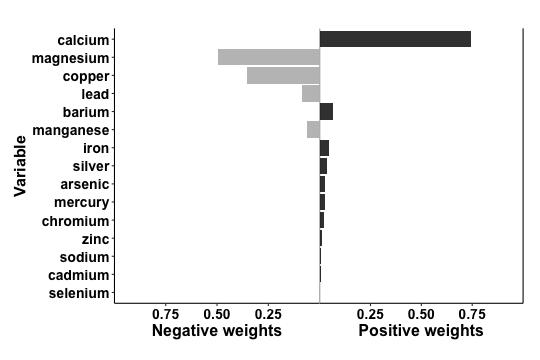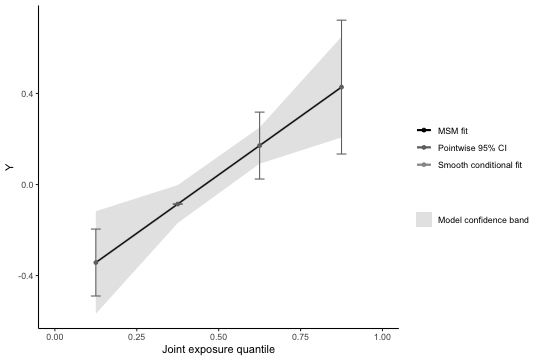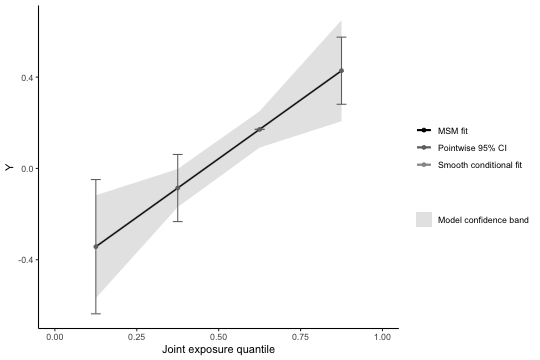From the first plot we see weights from `qgcomp.noboot` function, which include both positive and negative effect directions. When the weights are all on a single side of the null, these plots are easy to in interpret since the weight corresponds to the proportion of the overall effect from each exposure. WQS uses a constraint in the model to force all of the weights to be in the same direction - unfortunately such constraints lead to biased effect estimates. The `qgcomp` package takes a different approach and allows that “weights” might go in either direction, indicating that some exposures may beneficial, and some harmful, or there may be sampling variation due to using small or moderate sample sizes (or, more often, systematic bias such as unmeasured confounding). The “weights” in `qgcomp` correspond to the proportion of the overall effect when all of the exposures have effects in the same direction, but otherwise they correspond to the proportion of the effect in a particular direction, which may be small (or large) compared to the overall “mixture” effect. NOTE: the left and right sides of the plot should not be compared with each other because the length of the bars corresponds to the effect size only relative to other effects in the same direction. The darkness of the bars corresponds to the overall effect size - in this case the bars on the right (positive) side of the plot are darker because the overall “mixture” effect is positive. Thus, the shading allows one to make informal comparisons across the left and right sides: a large, darkly shaded bar indicates a larger independent effect than a large, lightly shaded bar.

Using `qgcomp.boot` also allows us to assess linearity of the total exposure effect (the second plot). Similar output is available for WQS (`gWQS` package), though WQS results will generally be less interpretable when exposure effects are non-linear (see below how to do this with `qgcomp.boot`).

The plot for the `qcboot.fit3` object (using g-computation with bootstrap variance) gives predictions at the joint intervention levels of exposure. It also displays a smoothed (graphical) fit. Generally, we cannot overlay the data over this plot since the regression line corresponds to a change in potentially many exposures at once. Hence, it is useful to explore non-linearity by fitting models that allow for non-linear effects.

### Example 4: non-linearity (and non-homogeneity)

`qgcomp` (and `qgcomp.boot`) addresses non-linearity in a way similar to standard parametric regression models, which lends itself to being able to leverage R language features for non-linear parametric models (or, more precisely, parametric models that deviate from a purely additive, linear function on the link function basis via the use of basis function representation of non-linear functions). Here is an example where we use a feature of the R language for fitting models with interaction terms. We use `y~. + .^2` as the model formula, which fits a model that allows for quadratic term for every predictor in the model.

Similar approaches could be used to include interaction terms between exposures, as well as between exposures and covariates.

``````qcboot.fit4 <- qgcomp(y~. + .^2,
expnms=Xnm,
metals[,c(Xnm, 'y')], family=gaussian(), q=4, B=10, seed=125)
plot(qcboot.fit4)
``````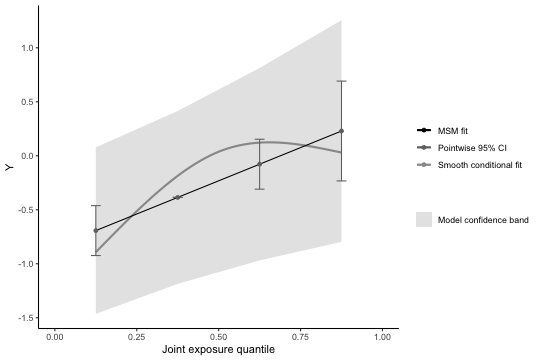Note that allowing for a non-linear effect of all exposures induces an apparent non-linear trend in the overall exposure effect. The smoothed regression line is still well within the confidence bands of the marginal linear model (by default, the overall effect of joint exposure is assumed linear, though this assumption can be relaxed via the 'degree' parameter in qgcomp.boot, as follows:

``````qcboot.fit5 <- qgcomp(y~. + .^2,
expnms=Xnm,
metals[,c(Xnm, 'y')], family=gaussian(), q=4, degree=2,
B=10, rr=FALSE, seed=125)
qgcomp::pointwisebound.boot(qcboot.fit5)
``````
``````##   quantile quantile.midpoint     linpred mean.diff   se.diff    ll.diff
## 0        0             0.125 -0.89239044 0.0000000 0.0000000  0.0000000
## 1        1             0.375 -0.18559680 0.7067936 0.6165306 -0.5015841
## 2        2             0.625  0.12180659 1.0141970 0.6044460 -0.1704954
## 3        3             0.875  0.02981974 0.9222102 0.3537861  0.2288022
##    ul.diff ll.linpred ul.linpred
## 0 0.000000 -0.8923904 -0.8923904
## 1 1.915171 -1.3939746  1.0227810
## 2 2.198889 -1.0628859  1.3064991
## 3 1.615618 -0.6635882  0.7232277
``````
``````qgcomp:::modelbound.boot(qcboot.fit5)
``````
``````##   quantile quantile.midpoint     linpred           m      se.pw      ll.pw
## 0        0             0.125 -0.89239044 -0.89239044 0.70335801 -2.2709468
## 1        1             0.375 -0.18559680 -0.18559680 0.09874085 -0.3791253
## 2        2             0.625  0.12180659  0.12180659 0.14624914 -0.1648365
## 3        3             0.875  0.02981974  0.02981974 0.83609757 -1.6089014
##         ul.pw    ll.simul    ul.simul
## 0 0.486165922 -2.08813110  0.18308760
## 1 0.007931712 -0.32577422 -0.02886665
## 2 0.408449640 -0.06371183  0.43431431
## 3 1.668540859 -1.19007238  1.57263047
``````
``````plot(qcboot.fit5)
``````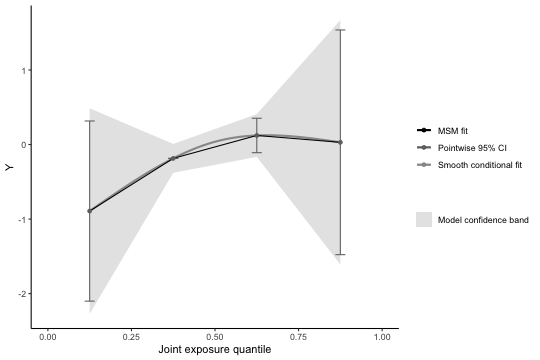Ideally, the smooth fit will look very similar to the model prediction regression line.

### Example 5: Comparing model fits and further exploring non-linearity

Exploring a non-linear fit in settings with multiple exposures is challenging. One way to explore non-linearity, as demonstrated above, is to to include all 2-way interaction terms (including quadratic terms, or “self-interactions”). Sometimes this approach is not desired, either because the number of terms in the model can become very large, or because some sort of model selection procedure is required, which risks inducing over-fit (biased estimates and standard errors that are too small). Short of having a set of a priori non-linear terms to include, we find it best to take a default approach (e.g. taking all second order terms) that doesn't rely on statistical significance, or to simply be honest that the search for a non-linear model is exploratory and shouldn't be relied upon for robust inference. Methods such as kernel machine regression may be good alternatives, or supplementary approaches to exploring non-linearity.

NOTE: qgcomp necessarily fits a regression model with exposures that have a small number of possible values, based on the quantile chosen. By package default, this is `q=4`, but it is difficult to fully examine non-linear fits using only four points, so we recommend exploring larger values of `q`, which will change effect estimates (i.e. the model coefficient implies a smaller change in exposures, so the expected change in the outcome will also decrease).

Here, we examine a one strategy for default and exploratory approaches to mixtures that can be implemented in qgcomp using a smaller subset of exposures (iron, lead, cadmium), which we choose via the correlation matrix. High correlations between exposures may result from a common source, so small subsets of the mixture may be useful for examining hypotheses that relate to interventions on a common environmental source or set of behaviors. Note that we can still adjust for the measured exposures, even though only 3 our exposures of interest are considered as the mixture of interest. Note that we will require a new R package to help in exploring non-linearity: `splines`. Note that `qgcomp.boot` must be used in order to produce the graphics below, as `qgcomp.noboot` does not calculate the necessary quantities.

#### Graphical approach to explore non-linearity in a correlated subset of exposures using splines

``````library(splines)
# find all correlations > 0.6 (this is an arbitrary choice)
cormat = cor(metals[,Xnm])
idx = which(cormat>0.6 & cormat <1.0, arr.ind = TRUE)

mage35 + arsenic + magnesium + manganese + mercury +
selenium + silver + sodium + zinc,
expnms=newXnm,
metals, family=gaussian(), q=8, B=10)

mage35 + arsenic + magnesium + manganese + mercury +
selenium + silver + sodium + zinc,
expnms=newXnm,
metals, family=gaussian(), q=8, B=10, degree=2)

mage35 + arsenic + magnesium + manganese + mercury +
selenium + silver + sodium + zinc,
expnms=newXnm,
metals, family=gaussian(), q=8, B=10, degree=3)
# it helps to place the plots on a common y-axis, which is easy due
#  to dependence of the qgcomp plotting functions on ggplot
pl.fit6lin <- plot(qc.fit6lin, suppressprint = TRUE, pointwiseref = 4)
pl.fit6nonlin <- plot(qc.fit6nonlin, suppressprint = TRUE, pointwiseref = 4)
pl.fit6nonhom <- plot(qc.fit6nonhom, suppressprint = TRUE, pointwiseref = 4)

pl.fit6lin + coord_cartesian(ylim=c(-0.75, .75)) +
pl.fit6nonlin + coord_cartesian(ylim=c(-0.75, .75)) +
pl.fit6nonhom + coord_cartesian(ylim=c(-0.75, .75)) +
``````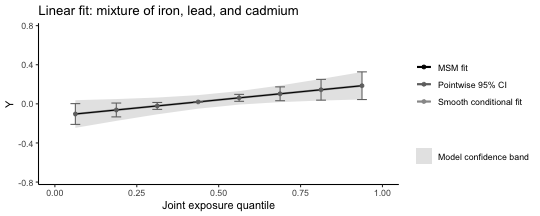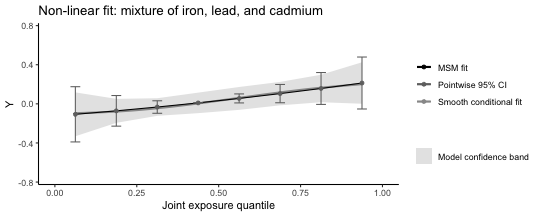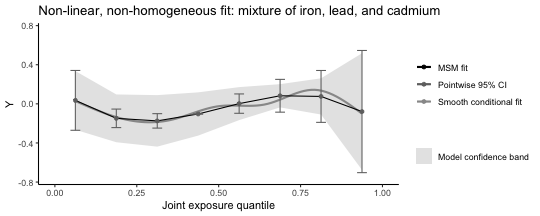The underlying conditional model fit can be made extremely flexible, and the graphical representation of this (via the smooth conditional fit) can look extremely flexible. Simply matching the overall (MSM) fit to this line is not a viable strategy for identifying parsimonious models because that would ignore potential for overfit. Thus, caution should be used when judging the accuracy of a fit when comparing the “smooth conditional fit” to the “MSM fit.” Here, there is little statistical evidence for even a linear trend, which makes the smoothed conditional fit appear to be overfit. The smooth conditional fit can be turned off, as below.

``````qc.overfit <- qgcomp.boot(y ~ bs(iron) + bs(cadmium) + bs(lead) +
mage35 + bs(arsenic) + bs(magnesium) + bs(manganese) + bs(mercury) +
bs(selenium) + bs(silver) + bs(sodium) + bs(zinc),
expnms=Xnm,
metals, family=gaussian(), q=8, B=10, degree=1)
qc.overfit
``````
``````## Mixture slope parameters (bootstrap CI):
##
##              Estimate Std. Error  Lower CI Upper CI t value Pr(>|t|)
## (Intercept) -0.064420   0.123768 -0.307001 0.178162 -0.5205   0.6030
## psi1         0.029869   0.031972 -0.032795 0.092534  0.9342   0.3507
``````
``````plot(qc.overfit, pointwiseref = 5)
plot(qc.overfit, flexfit = FALSE, pointwiseref = 5)
``````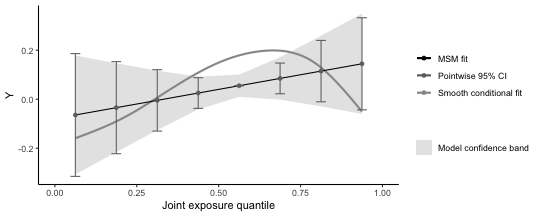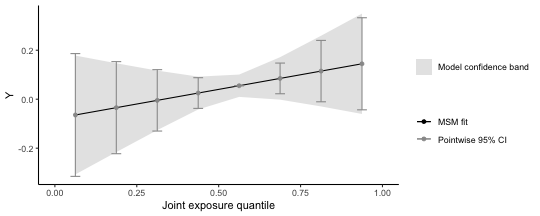### Example 6: Miscellaneous other ways to allow non-linearity.

Note that these are included as examples of how to include non-linearities, and are not intended as a demonstration of appropriate model selection. In fact, qc.fit7b is generally a bad idea in small to moderate sample sizes due to large numbers of parameters.

``````# using indicator terms for each quantile
mage35 + arsenic + magnesium + manganese + mercury +
selenium + silver + sodium + zinc,
expnms=newXnm,
metals, family=gaussian(), q=8, B=20, deg=2)
# underlying fit
summary(qc.fit7a\$fit)\$coefficients
``````
``````##                   Estimate Std. Error    t value     Pr(>|t|)
## (Intercept)   -0.052981109 0.08062430 -0.6571357 5.114428e-01
## factor(iron)1  0.046571725 0.09466914  0.4919420 6.230096e-01
## factor(iron)2 -0.056984300 0.09581659 -0.5947227 5.523395e-01
## factor(iron)3  0.143813131 0.09558055  1.5046276 1.331489e-01
## factor(iron)4  0.053319057 0.09642069  0.5529835 5.805601e-01
## factor(iron)5  0.303967859 0.09743261  3.1197753 1.930856e-03
## factor(iron)6  0.246259568 0.09734385  2.5297907 1.176673e-02
## factor(iron)7  0.447045591 0.09786201  4.5681217 6.425565e-06
## lead          -0.009210341 0.01046668 -0.8799679 3.793648e-01
## cadmium       -0.010503041 0.01086440 -0.9667387 3.342143e-01
## mage35         0.081114695 0.07274583  1.1150426 2.654507e-01
## arsenic        0.021755516 0.02605850  0.8348720 4.042502e-01
## magnesium     -0.010758356 0.02469893 -0.4355798 6.633587e-01
## manganese      0.004418266 0.02551449  0.1731670 8.626011e-01
## mercury        0.003913896 0.02448078  0.1598763 8.730531e-01
## selenium      -0.058085344 0.05714805 -1.0164012 3.100059e-01
## silver         0.020971562 0.02407397  0.8711302 3.841658e-01
## sodium        -0.062086322 0.02404447 -2.5821454 1.014626e-02
## zinc           0.017078438 0.02392381  0.7138679 4.756935e-01
``````
``````plot(qc.fit7a)

# interactions between indicator terms
mage35 + arsenic + magnesium + manganese + mercury +
selenium + silver + sodium + zinc,
expnms=newXnm,
metals, family=gaussian(), q=8, B=10, deg=3)
# underlying fit
#summary(qc.fit7b\$fit)\$coefficients
plot(qc.fit7b)

# breaks at specific quantiles (these breaks act on the quantized basis)
mage35 + arsenic + magnesium + manganese + mercury +
selenium + silver + sodium + zinc,
expnms=newXnm,
metals, family=gaussian(), q=8, B=10, deg=2)
# underlying fit
summary(qc.fit7c\$fit)\$coefficients
``````
``````##                                      Estimate Std. Error      t value
## (Intercept)                     -5.910113e-02 0.05182385 -1.140423351
## I(iron > 4)TRUE                  3.649940e-01 0.06448858  5.659824144
## I(lead > 4)TRUE                 -9.004067e-05 0.06181587 -0.001456595
## mage35                           7.613672e-02 0.07255110  1.049422029
## arsenic                          2.042370e-02 0.02578001  0.792230124
## magnesium                       -3.279980e-03 0.02427513 -0.135116878
## manganese                        1.055979e-02 0.02477453  0.426235507
## mercury                          9.396898e-03 0.02435057  0.385900466
## selenium                        -4.337729e-02 0.05670006 -0.765030761
## silver                           1.807248e-02 0.02391112  0.755819125
## sodium                          -5.537968e-02 0.02403808 -2.303831424
## zinc                             2.349906e-02 0.02385762  0.984970996
## I(iron > 4)TRUE:I(lead > 4)TRUE -1.828835e-01 0.10277790 -1.779405131
##                                     Pr(>|t|)
## (Intercept)                     2.547332e-01
## I(iron > 4)TRUE                 2.743032e-08
## mage35                          2.945626e-01
## arsenic                         4.286554e-01
## magnesium                       8.925815e-01
## manganese                       6.701456e-01
## mercury                         6.997578e-01
## selenium                        4.446652e-01
## silver                          4.501639e-01
## sodium                          2.169944e-02
## zinc                            3.251821e-01
## I(iron > 4)TRUE:I(lead > 4)TRUE 7.586670e-02
``````
``````plot(qc.fit7c)
``````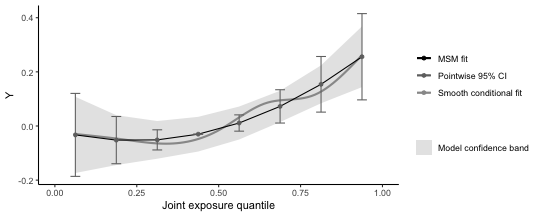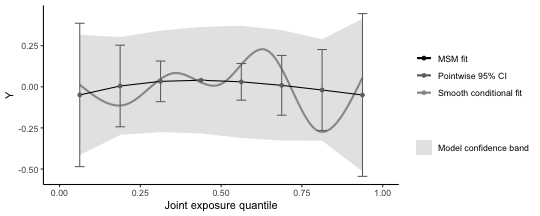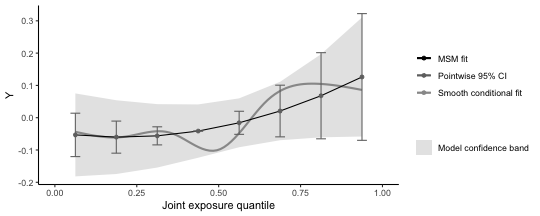Note one restriction on exploring non-linearity: while we can use flexible functions such as splines for individual exposures, the overall fit is limited via the `degree` parameter to polynomial functions (here a quadratic polynomial fits the non-linear model well, and a cubic polynomial fits the non-linear/non-homogenous model well - though this is an informal argument and does not account for the wide confidence intervals). We note here that only 10 bootstrap iterations are used to calculate confidence intervals (to increase computational speed for the example), which is far too low.

#### Statistical approach explore non-linearity in a correlated subset of exposures using splines

The graphical approaches don't give a clear picture of which model might be preferred, but we can compare the model fits using AIC, or BIC (information criterion that weigh model fit with over-parameterization). Both of these criterion suggest the linear model fits best (lowest AIC and BIC), which suggests that the apparently non-linear fits observed in the graphical approaches don't improve prediction of the health outcome, relative to the linear fit, due to the increase in variance associated with including more parameters.

``````AIC(qc.fit6lin\$fit)
``````
``````##  676.0431
``````
``````AIC(qc.fit6nonlin\$fit)
``````
``````##  682.7442
``````
``````AIC(qc.fit6nonhom\$fit)
``````
``````##  705.6187
``````
``````BIC(qc.fit6lin\$fit)
``````
``````##  733.6346
``````
``````BIC(qc.fit6nonlin\$fit)
``````
``````##  765.0178
``````
``````BIC(qc.fit6nonhom\$fit)
``````
``````##  898.9617
``````

### Example 7: time-to-event analysis and parallel processing

• The `qgcomp` package utilizes Cox proportional hazards models as the underlying model for time-to-event analysis. The interpretation of a `qgcomp.noboot` fit parameter is a conditional (on confounders) hazard ratio for increasing all exposures at once. The `qc.survfit1` object demonstrates a time-to- event analysis with `qgcompcox.noboot`. The default plot is similar to that of `qgcompcox.noboot`, in that it yields weights and an overall mixture effect
``````# non-bootstrapped version estimates a marginal structural model for the
# confounder-conditional effect
arsenic + magnesium + manganese + mercury +
selenium + silver + sodium + zinc +
mage35,
data=metals)
``````
``````## Call:
## survival::coxph(formula = survival::Surv(disease_time, disease_state) ~
##     iron + lead + cadmium + arsenic + magnesium + manganese +
##         mercury + selenium + silver + sodium + zinc + mage35,
##     data = metals)
##
##                coef exp(coef)  se(coef)      z      p
## iron      -0.056447  0.945117  0.156178 -0.361 0.7178
## lead       0.440735  1.553849  0.203264  2.168 0.0301
## cadmium    0.023325  1.023599  0.105502  0.221 0.8250
## arsenic   -0.003812  0.996195  0.088897 -0.043 0.9658
## magnesium  0.099399  1.104507  0.064730  1.536 0.1246
## manganese -0.014065  0.986033  0.064197 -0.219 0.8266
## mercury   -0.060830  0.940983  0.072918 -0.834 0.4042
## selenium  -0.231626  0.793243  0.173655 -1.334 0.1823
## silver     0.043169  1.044114  0.070291  0.614 0.5391
## sodium     0.057928  1.059638  0.063883  0.907 0.3645
## zinc       0.057169  1.058835  0.047875  1.194 0.2324
## mage35    -0.458696  0.632107  0.238370 -1.924 0.0543
##
## Likelihood ratio test=23.52  on 12 df, p=0.02364
## n= 452, number of events= 205
``````
``````qc.survfit1 <- qgcomp.cox.noboot(survival::Surv(disease_time, disease_state) ~ .,expnms=Xnm,
data=metals[,c(Xnm, 'disease_time', 'disease_state')], q=4)
qc.survfit1
``````
``````## Scaled effect size (positive direction, sum of positive coefficients = 0.32)
##    barium      zinc magnesium  chromium    silver    sodium      iron
##    0.3432    0.1946    0.1917    0.1119    0.0924    0.0511    0.0151
##
## Scaled effect size (negative direction, sum of negative coefficients = -0.554)
##    0.2705    0.1826    0.1666    0.1085    0.0974    0.0794    0.0483    0.0466
##
## Mixture log(hazard ratio) (Delta method CI):
##
##      Estimate Std. Error Lower CI Upper CI Z value Pr(>|z|)
## psi1 -0.23356    0.24535 -0.71444  0.24732 -0.9519   0.3411
``````
``````plot(qc.survfit1)
``````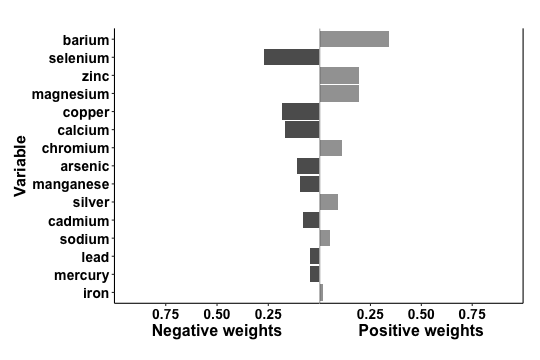Marginal hazards ratios (and bootstrapped quantile g-computation in general) uses a slightly different approach to effect estimation that makes it more computationally demanding than other `qcomp` functions.

To estimate a marginal hazards ratio, the underlying model is fit, and then new outcomes are simulated under the underlying model with a baseline hazard estimator (Efron's) - this simulation requires a large sample (controlled by MCsize) for accuracy. This approach is similar to other g-computation approaches to survival analysis, but this approach uses the exact survival times, rather than discretized survival times as are common in most g-computation analysis. Plotting a `qgcompcox.boot`object yields a set of survival curves (e.g.`qc.survfit2`) which comprise estimated survival curves (assuming censoring and late entry at random, conditional on covariates in the model) that characterize conditional survival functions (i.e. censoring competing risks) at various levels of joint-exposure (including the overall average - which may be slightly different from the observed survival curve, but should more or less agree).

``````# bootstrapped version estimates a marginal structural model for the population average effect
#library(survival)
qc.survfit2 <- qgcomp.cox.boot(Surv(disease_time, disease_state) ~ .,expnms=Xnm,
data=metals[,c(Xnm, 'disease_time', 'disease_state')], q=4,
B=5, MCsize=1000, parallel=TRUE)
qc.survfit2
``````
``````## Mixture log(hazard ratio) (bootstrap CI):
##
##      Estimate Std. Error Lower CI Upper CI Z value Pr(>|z|)
## psi1 -0.24020    0.14407 -0.52257 0.042168 -1.6673  0.09546
``````
``````# testing proportional hazards (note that x=TRUE is not needed (and will cause an error if used))
survival::cox.zph(qc.survfit2\$fit)
``````
``````##             chisq df     p
## arsenic   0.18440  1 0.668
## barium    0.10819  1 0.742
## calcium   0.00206  1 0.964
## chromium  1.23974  1 0.266
## copper    0.28518  1 0.593
## iron      3.46739  1 0.063
## magnesium 2.12900  1 0.145
## manganese 0.58720  1 0.444
## mercury   0.00136  1 0.971
## selenium  0.15247  1 0.696
## silver    0.01040  1 0.919
## sodium    0.09352  1 0.760
## zinc      1.51261  1 0.219
## GLOBAL    9.82045 15 0.831
``````
``````p2 = plot(qc.survfit2, suppressprint = TRUE)
p2 + labs(title="Linear log(hazard ratio), overall and exposure specific")
``````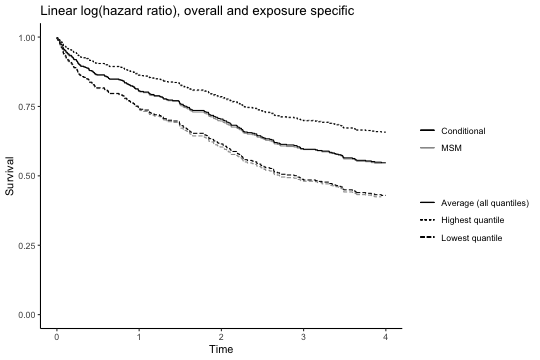All bootstrapped functions in `qgcomp` allow parellelization via the parallel=TRUE parameter (demonstrated with the non-liner fit in `qc.survfit3`). Only 5 bootstrap iterations are used here, which is not nearly enough for inference, and will actually be slower for parallel processing due to some overhead when setting up the parallel processes.

``````qc.survfit3 <- qgcomp.cox.boot(Surv(disease_time, disease_state) ~ . + .^2,expnms=Xnm,
data=metals[,c(Xnm, 'disease_time', 'disease_state')], q=4,
B=5, MCsize=1000, parallel=TRUE)
qc.survfit3
``````
``````## Mixture log(hazard ratio) (bootstrap CI):
##
##      Estimate Std. Error Lower CI Upper CI Z value Pr(>|z|)
## psi1 -0.19766    0.89556  -1.9529   1.5576 -0.2207   0.8253
``````
``````p3 = plot(qc.survfit3, suppressprint = TRUE)
p3 + labs(title="Non-linear log(hazard ratio) overall, linear exposure specific ln-HR")
``````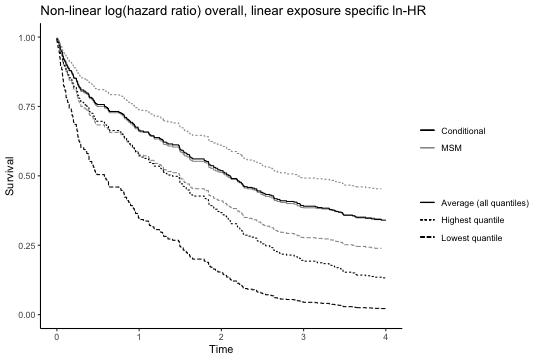While `qgcompcox.boot` fits a smooth hazard ratio function, the hazard ratios contrasting specific quantiles with a referent quantile can be obtained, as demonstrated with `qc.survfit4`. As in `qgcomp.boot` plots, the conditional model fit and the MSM fit are overlaid as a way to judge how well the MSM fits the conditional fit (and whether, for example non-linear terms should be added or removed from the overall fit via the degree parameter - we note here that we know of no statistical test for quantifying the difference between these lines, so this is up to user discretion and the plots are provided as visuals to aid in exploratory data analysis).

``````qc.survfit4 <- qgcomp.cox.boot(Surv(disease_time, disease_state) ~ . + .^2,expnms=Xnm,
data=metals[,c(Xnm, 'disease_time', 'disease_state')], q=4,
B=5, MCsize=1000, parallel=TRUE, degree=2)
qc.survfit4
``````
``````## Mixture log(hazard ratio) (bootstrap CI):
##
##      Estimate Std. Error Lower CI Upper CI Z value Pr(>|z|)
## psi1 -2.87367    4.34082 -11.3815   5.6342 -0.6620   0.5080
## psi2  0.87788    1.56189  -2.1834   3.9391  0.5621   0.5741
``````
``````# examining the overall hazard ratio as a function of overall exposure
hrs_q = exp(matrix(c(0,0,1,1,2,4,3,9), ncol=2, byrow=TRUE)%*%qc.survfit4\$msmfit\$coefficients)
colnames(hrs_q) = "Hazard ratio"
print("Hazard ratios by quartiles (min-25%,25-50%, 50-75%, 75%-max)")
``````
``````##  "Hazard ratios by quartiles (min-25%,25-50%, 50-75%, 75%-max)"
``````
``````hrs_q
``````
``````##      Hazard ratio
## [1,]    1.0000000
## [2,]    0.1359070
## [3,]    0.1069065
## [4,]    0.4867284
``````
``````p4 = plot(qc.survfit4, suppressprint = TRUE)
p4 + labs(title="Non-linear log(hazard ratio), overall and exposure specific")
``````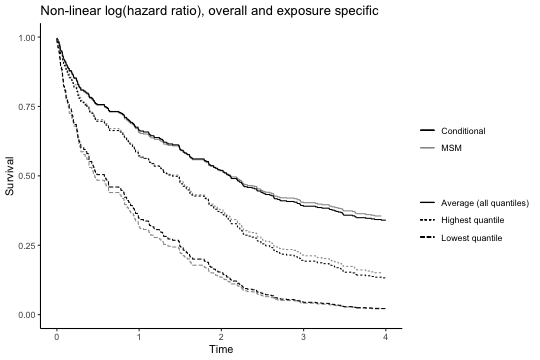Testing proportional hazards is somewhat complicated with respect to interpretation. Consider first a linear fit from `qgcomp.cox.noboot`. Because the underlying model of a linear qgcomp fit is equivalent to the sum of multiple parameters, it is not clear how proportional hazards might be best tested for the mixture. One could examine test statistics for each exposure, but there may be some exposures for which the test indicates non-proportional hazards and some for which the test does not.

The “GLOBAL” test in the cox.zph function from the `survival` package comes closest to what we might want, and gives an overall assessment of non-proportional hazards for all model terms simultaneously (including non-mixture covariates). While this seems somewhat undesirable due to non-specificity, it is not necessarily important that only the mixture have proportional hazards, so it is useful and easily interpretable to have a global test of fit via GLOBAL.

``````# testing proportional hazards (must set x=TRUE in function call)
qc.survfit1ph <- qgcomp.cox.noboot(survival::Surv(disease_time, disease_state) ~ .,expnms=Xnm,
data=metals[,c(Xnm, 'disease_time', 'disease_state', "mage35")], q=4,
x=TRUE)
survival::cox.zph(qc.survfit1ph\$fit)
``````
``````##              chisq df     p
## arsenic   1.57e-01  1 0.691
## barium    1.28e-01  1 0.721
## calcium   9.16e-04  1 0.976
## chromium  1.25e+00  1 0.263
## copper    3.42e-01  1 0.559
## iron      3.51e+00  1 0.061
## magnesium 2.08e+00  1 0.149
## manganese 5.78e-01  1 0.447
## mercury   4.87e-06  1 0.998
## selenium  1.32e-01  1 0.716
## silver    1.30e-02  1 0.909
## sodium    1.06e-01  1 0.745
## zinc      1.53e+00  1 0.216
## mage35    1.73e-02  1 0.895
## GLOBAL    9.93e+00 16 0.870
``````

For a potentially non-linear/ non-additive fit from `qgcomp.cox.boot`, the issue is slightly more complicated by the fact that the algorithm will fit both the underlying model and a marginal structural model using the predictions from the underlying model. In order for the predictions to yield valid causal inference, the underlying model must be correct (which implies that proportional hazards hold). The marginal structural model proceeds assuming the underlying model is correct. Currently there is no simple way to allow for non-proportional hazards in the marginal structural model, but non-proportional hazards can be implemented in the conditional model via standard approaches to non-proportional hazards such as time-exposure-interaction terms. This is a rich field and discussion is beyond the scope of this document.

``````# testing global proportional hazards for model (note that x=TRUE is not needed (and will cause an error if used))
phtest3 = survival::cox.zph(qc.survfit3\$fit)
phtest3\$table[dim(phtest3\$table),, drop=FALSE]
``````
``````##           chisq  df           p
## GLOBAL 206.5578 120 1.53907e-06
``````

Late entry and counting-process style data will currently yield results in qgcomp.cox functions. There has been some testing of this in limited settings, but we note that this is still an experimental feature at this point that may not be valid in all cases and so it is not documented here. As much effort as possible to validate results through other means is needed when using qgcomp in data subject to late-entry or when using counting-process style data.

### Example 8: clustering

Clustering on the individual or group level means that there are individual or group level characteristics which result in covariance between observations (e.g. within individual variance of an outcome may be much lower than the between individual variance). For linear models, the error term is assumed to be independent between observations, and clustering breaks this assumption. Ways to relax this assumption include empirical variance estimation and cluster-appropriate robust variance estimation (e.g. through the `sandwich` package in R). Another way is to use cluster-based bootstrapping, which samples clusters, rather than individual observations. `qgcomp.boot` can be leveraged to produce clustering consistent estimates of standard errors for independent effects of exposure as well as the effect of the exposure as a whole. This is done using the `id` parameter of `qgcomp.boot` (which can only handle a single variable and so may not efficient for nested clustering, for example).

Below is a simple example with one simulated exposure. First the exposure data are 'pre-quantized' (so that one can verify that standard errors are appropriate using other means - this is just to prove that the clustering works and is not intended to show a suggested practice). Next the data are analyzed using a 1-component mixture in qgcomp - again, this is for verification purposes. The `qgcomp.noboot` result yields a naive standard error of 0.0310 for the mixture effect, while the `qgcomp.boot` result (MCsize=5000, B=500) yields a corrected standard error of 0.0398, which is much closer to the sandwich estimate of 0.0409 than the naive estimator (a second `qgcomp.boot` fit with fewer bootstrap iterations and smaller MCsize is included for display, but the more accurate results are commented out to reduce runtime). Here, the standard errors from the uncorrected fit are too low, but this may not always be the case.

``````set.seed(2123)
N = 250
t = 4
dat <- data.frame(row.names = 1:(N*t))
dat <- within(dat, {
id = do.call("c", lapply(1:N, function(x) rep(x, t)))
u =  do.call("c", lapply(1:N, function(x) rep(runif(1), t)))
x1 = rnorm(N, u)
y = rnorm(N) + u + x1
})

# pre-quantize
expnms = c("x1")
datl = quantize(dat, expnms = expnms)

qgcomp.noboot(y~ x1, data=datl\$dat, family=gaussian(), q = NULL)
``````
``````## Including all model terms as exposures of interest
``````
``````## Scaled effect size (positive direction, sum of positive coefficients = 0.955)
## x1
##  1
##
## Scaled effect size (negative direction, sum of negative coefficients = 0)
## None
##
## Mixture slope parameters (Delta method CI):
##
##              Estimate Std. Error Lower CI Upper CI t value  Pr(>|t|)
## (Intercept) -0.463243   0.057934 -0.57679 -0.34969  -7.996 3.553e-15
## psi1         0.955015   0.031020  0.89422  1.01581  30.787 < 2.2e-16
``````
``````# neither of these ways yields appropriate clustering
#qgcomp.noboot(y~ x1, data=datl\$dat, id="id", family=gaussian(), q = NULL)
#qgcomp.boot(y~ x1, data=datl\$dat, family=gaussian(), q = NULL, MCsize=1000)

# clustering by specifying id parameter on
qgcomp.boot(y~ x1, data=datl\$dat, id="id", family=gaussian(), q = NULL, MCsize=1000, B = 5)
``````
``````## Including all model terms as exposures of interest
``````
``````##
## Note: using all possible values of exposure as the
##               intervention values
``````
``````## Mixture slope parameters (bootstrap CI):
##
##              Estimate Std. Error Lower CI Upper CI t value  Pr(>|t|)
## (Intercept) -0.463243   0.084446 -0.62875 -0.29773 -5.4856 5.223e-08
## psi1         0.955015   0.055037  0.84714  1.06289 17.3521 < 2.2e-16
``````
``````#qgcomp.boot(y~ x1, data=datl\$dat, id="id", family=gaussian(), q = NULL, MCsize=1000, B = 500)
#   Mixture slope parameters (bootstrap CI):
#
#               Estimate Std. Error Lower CI Upper CI t value
#   (Intercept)  -0.4632     0.0730   -0.606    -0.32 3.3e-10
#   psi1          0.9550     0.0398    0.877     1.03       0

# This can be verified using the `sandwich` package
#fitglm = glm(y~x1, data=datl\$dat)
#sw.cov = sandwich::vcovCL(fitglm, cluster=~id, type = "HC0")[2,2]
#sqrt(sw.cov)
#  0.0409
``````

### Example 9: partial effects

Returning to our original example (and adjusting for covariates), note that the default output for a qgcomp.*.noboot object includes “sum of positive/negative coefficients.” These can be interpreted as “partial mixture effects” or effects of exposures with coefficients in a particular direction. This is displayed graphically via a plot of the qgcomp “weights,” where all exposures that contribute to a given partial effect are on the same side of the plot. Unfortunately, this does not yield valid inference for a true partial effect because it is a parameter conditional on the fitted results and thus does not represent the type of a priori hypothesis that is amenable to hypothesis testing and confidence intervals. Another way to think about this is that it is a data adaptive parameter and thus is subject to issues of overfit that are similar to issues with making inference from step-wise variable selection procedures.

``````(qc.fit.adj <- qgcomp.noboot(y~.,dat=metals[,c(Xnm, covars, 'y')], expnms=Xnm, family=gaussian()))
``````
``````## Scaled effect size (positive direction, sum of positive coefficients = 0.441)
##  calcium   barium     iron  arsenic   silver chromium  cadmium     zinc
##  0.76111  0.06133  0.05854  0.02979  0.02433  0.02084  0.01395  0.01195
##  mercury   sodium
##  0.01130  0.00688
##
## Scaled effect size (negative direction, sum of negative coefficients = -0.104)
##    copper magnesium      lead manganese  selenium
##   0.44654   0.42124   0.09012   0.03577   0.00631
##
## Mixture slope parameters (Delta method CI):
##
##              Estimate Std. Error Lower CI Upper CI t value  Pr(>|t|)
## (Intercept) -0.460408   0.134007 -0.72306 -0.19776 -3.4357 0.0006477
## psi1         0.337539   0.089358  0.16240  0.51268  3.7774 0.0001805
``````
``````plot(qc.fit.adj)
``````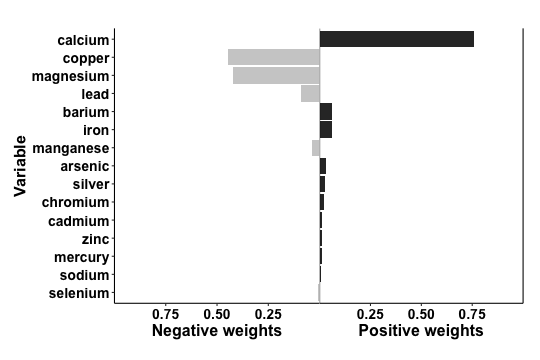Fortunately, there is a way towards estimation of “partial effects.” One way to do this is sample splitting, where the data are first randomly partitioned into a “training” and a “validation” data set. The assessment of whether a coefficient is positive or not occurs in the “training” set and then effect estimation for positive/negative partial effects occurs in the validation data. Basic simulations can show that such a procedure can yield valid (often called “honest”) hypothesis tests and confidence intervals for partial effects, provided that there is no separate data exploration in the combined dataset to select the models. In the `qgcomp` package, the partitioning of the datasets into “training” and “validation” sets is done by the user, which prevents issues that may arise if this is done naively on a dataset that contains clusters (where we should partition based on clusters, rather than observations) or multiple observations per individual (all observations from an individual should be partitioned together). Here is an example of simple partitioning on a dataset that contains one observation per individual with no clustering. The downside of sample splitting is the loss of precision, because the final “validation” dataset comprises only a fraction of the original sample size. Thus, the estimation of partial effects is most appropriate with large sample sizes. We also note that these partial effect are only well defined when all effects are linear and additive, since whether a variable contributes to a positive or negative partial effect would depend on the value of that variable, so the valid estimation of “partial effects” is limited to settings in which the `qgcomp.\*.noboot` objects are used for inference.

``````# 40/60% training/validation split
set.seed(1231124)
trainidx <- sample(1:nrow(metals), round(nrow(metals)*0.4))
valididx <- setdiff(1:nrow(metals),trainidx)
traindata <- metals[trainidx,]
validdata <- metals[valididx,]
dim(traindata) # 181 observations = 40% of total
``````
``````##  181  26
``````
``````dim(validdata) # 271 observations = 60% of total
``````
``````##  271  26
``````

The qgcomp package then facilitates the analysis of these partitioned data to allow valid estimation and hypothesis testing of partial effects. The `qgcomp.partials` function is used to estimate partial effects. Note that the variables with “negative effect sizes” differs slightly from the overall analysis given in the `qc.fit` object that represents our first pass analysis on these data. This is to be expected, and is a feature of this approach: different random subsets of the data will be expected to yield different estimated effects. If the true effect is null, then the estimated effects will vary from positive to negative around the null, and sample splitting is an important way to distinguish between estimates that reflect underlying patterns in the entire dataset from estimates that are simply due to natural sampling variation inherent to small and moderate samples.

``````splitres <- qgcomp.partials(fun="qgcomp.noboot", f=y~., q=4,
traindata=traindata[,c(Xnm, covars, "y")],validdata=validdata[,c(Xnm, covars, "y")], expnms=Xnm)
splitres
``````
``````##
## Variables with positive effect sizes in training data: calcium, mercury, iron, barium, cadmium, silver, chromium, manganese, zinc
## Variables with negative effect sizes in training data: copper, sodium, arsenic, selenium, magnesium, lead
## Partial effect sizes estimated in validation data
## Positive direction Mixture slope parameters (Delta method CI):
##
##              Estimate Std. Error Lower CI Upper CI t value Pr(>|t|)
## (Intercept) -0.416523   0.148975 -0.70851 -0.12454 -2.7959 0.005560
## psi1         0.314599   0.098888  0.12078  0.50842  3.1814 0.001643
##
## Negative direction Mixture slope parameters (Delta method CI):
##
##              Estimate Std. Error Lower CI Upper CI t value Pr(>|t|)
## (Intercept)  0.072880   0.111731 -0.14611  0.29187  0.6523   0.5148
## psi1        -0.022265   0.073428 -0.16618  0.12165 -0.3032   0.7620
``````
``````plot(splitres\$pos.fit)
``````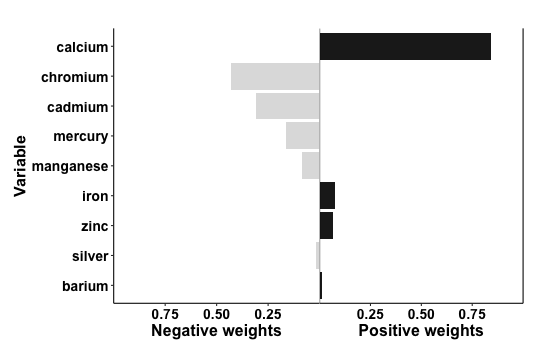Consistent with our overall results, the overall effect of metals on the simulated outcome `y` is driven by a positive effects from metals driven mainly by calcium. The “partial positive effect of psi=0.31 is attenuated from the [partial positive effect given in the original fit (0.44), but is similar to the overall effect from the original fit (psi1=0.34), which reflects the fact that the original partial effect estimates will be subject to more extreme values due to overfit, but the overall fit will not be as sensitive to overfit and validly estimates the partial effect in the setting where the effects of exposure are all in the same direction. We note that the effect directions of chromium, cadmium, mercury, silver, and manganese are negative, even though these were selected based on positive associations in the training data. This suggests these variables have effects that are close to the null and their direction will depend on which subset of the data are used. This feature allows valid testing of hypotheses - a `global null` in which no exposures have effects will be characterized by variables that randomly switch effect directions between training and validation datasets, which will yield partial effect estimates close to the null with large p-values.

One careful note: when there are multiple exposures with small positive or negative effects, the partial effects may be biased towards the null in studies with moderate or small sample sizes. This occurs because, in the training set, some exposures with small effects are likely to be misclassified with regard to their effect direction. This means that small negative effects can be added to large positive effects to yield smaller positive effects, and vice versa. In some instances, both the positive and negative partial effects can be in the same direction. This occurs if individual effects are predominantly in one direction, but some are small and subject to having misclassified directions. The sum of the partial positive and partial negative effects will still be close to the overall effect (where the difference would be due to sampling error), but there may be bias towards the null of the partial effects.

The larger question about interpretation (and its worth) of partial effects is left to the analyst. For large datasets with well characterized exposures that have plausible subsets of exposures that would be positively/negatively linearly associated with the outcome, the variables that partition into negative/positive partial effects may make some substantive sense. In more realistic settings that typify exposure mixtures, the partitioning will result in groups that don't entirely make sense. The "partial effect” yields the effect of increasing all exposures in the subset defined by positive coefficients in the training data, while holding all other exposures and confounders constant. In the setting where this corresponds to real world correlation patterns (e.g. all exposures in the positive partial effect share a source), then this may be interpretable roughly as an action to intervene on the source of these exposures. In most settings, however, interpretation will not be this clear and should not be expected to map onto potential real-world interventions. We note that this is not a function of the quantile g-computation method, but just part of the general messiness of working with exposures mixture data.

A more justifiable approach in terms of mapping effect estimates onto potential real-world actions would be choosing subsets of exposures based on prior subject matter knowledge, as we demonstrated above in example 5. This does not require sample splitting, but it does come at the expense of having to know more about the exposures and outcome under analysis. For example, our simulated outcome `y` may represent some outcome that we would expect to increase with so-called “essential” metals, or those that are necessary (at some small amount) for normal human functioning, but it may decrease with “potentially toxic” (non-essential) metals, or those that have no known biologic function and are more likely to cause harm rather than improve physiologic processes that lead to improved (larger) values of `y`. Qgcomp can be used to assess effects of these “submixtures.”

``````nonessentialXnm <- c(
)
essentialXnm <- c(
'sodium','magnesium','calcium','manganese','iron','copper','zinc','selenium'
)
covars = c('nitrate','nitrite','sulfate','ph', 'total_alkalinity','total_hardness')

(qc.fit.essential <- qgcomp.noboot(y~.,dat=metals[,c(Xnm, covars, 'y')], expnms=essentialXnm, family=gaussian()))
``````
``````## Scaled effect size (positive direction, sum of positive coefficients = 0.357)
##  calcium     iron     zinc selenium
## 0.908914 0.077312 0.013128 0.000646
##
## Scaled effect size (negative direction, sum of negative coefficients = -0.0998)
##    copper magnesium    sodium manganese
##    0.4872    0.4304    0.0486    0.0338
##
## Mixture slope parameters (Delta method CI):
##
##              Estimate Std. Error Lower CI Upper CI t value Pr(>|t|)
## (Intercept) -0.340130   0.111562 -0.55879 -0.12147 -3.0488 0.002435
## psi1         0.257136   0.074431  0.11125  0.40302  3.4547 0.000604
``````
``````(qc.fit.nonessential <- qgcomp.noboot(y~.,dat=metals[,c(Xnm, covars, 'y')], expnms=nonessentialXnm, family=gaussian()))
``````
``````## Scaled effect size (positive direction, sum of positive coefficients = 0.0751)
##  barium arsenic  silver mercury cadmium
##  0.2827  0.2494  0.2420  0.1763  0.0496
##
## Scaled effect size (negative direction, sum of negative coefficients = -0.0129)
##    0.888    0.112
##
## Mixture slope parameters (Delta method CI):
##
##              Estimate Std. Error  Lower CI Upper CI t value Pr(>|t|)
## (Intercept) -0.055549   0.081509 -0.215304  0.10420 -0.6815   0.4959
## psi1         0.062267   0.052508 -0.040648  0.16518  1.1858   0.2363
``````

As shown from these results, the essential metals and minerals demonstrate an overall positive joint association with the outcome (controlling for non-essentials), wheras the partial effect of non-essential metals and minerals is close to null. This is close to the interpretation of the data adaptive selection of partial effects demonstrated above, but is much more interpretable in terms of how we might intervene (e.g. increase consumption of foods that are higher in essential metals and minerals).

## Missing data, limits of detection and multiple imputation

When carrying out data analysis using quantile g-computation, on can address missing data in much the same way as in standard regression analyses. A common approach is complete case analysis. While regression functions in R will automatically carry out complete case analyses when variables take on the value `NA` (denoting missingness in R), when using quantile g-computation it is encouraged that one explicitly create the complete case dataset explicitly and use that complete case dataset. Using the pre-installed R packages, this can be accomplished with the `complete.cases` function.

The reason for this recommendation is that, while the regression analysis will be performed on complete cases (i.e. observations with non-missing values for all variables in the model), the calculation of quantiles for each exposures is done on an exposure-by-exposure basis, which can lead to numerical differences when explicitly using a dataset restricted to complete cases versus relying on automatically removing observations with `NA` values during analysis.

Here is an artificial example that demonstrates the differences. Three analyses are run: one with the full data, one with complete case data (complete case analysis #1), and one with data in which `arsenic` has been randomly set to `NA` (complete case analysis #2).

There are numeric differnces between the two complete case analyses, which can be traced to differences in the “quantized” exposures (other than arsenic) in the two approaches, which can be found by the `qx` data frame that is part of a `qgcompfit` object.

``````Xnm <- c(
'sodium','zinc'
)
covars = c('nitrate','nitrite','sulfate','ph', 'total_alkalinity','total_hardness')
asmiss = metals
set.seed(1232)
asmiss\$arsenic = ifelse(runif(nrow(metals))>0.7, NA, asmiss\$arsenic)
cc = asmiss[complete.cases(asmiss[,c(Xnm, covars, "y")]),] # complete.cases gives a logical index to subset rows
dim(metals) #  452  26
``````
``````##  452  26
``````
``````dim(cc) #  320  26
``````
``````##  320  26
``````
``````# complete case analysis
qc.base <- qgcomp.noboot(y~.,expnms=Xnm, dat=metals[,c(Xnm, covars, 'y')], family=gaussian())

qc.cc  <- qgcomp.noboot(y~.,expnms=Xnm, dat=cc[,c(Xnm, covars, 'y')], family=gaussian())

qc.cc2 <- qgcomp.noboot(y~.,expnms=Xnm, dat=asmiss[,c(Xnm, covars, 'y')], family=gaussian())

cat("Full data\n")
``````
``````## Full data
``````
``````qc.base
``````
``````## Scaled effect size (positive direction, sum of positive coefficients = 0.441)
##  calcium   barium     iron  arsenic   silver chromium  cadmium     zinc
##  0.76111  0.06133  0.05854  0.02979  0.02433  0.02084  0.01395  0.01195
##  mercury   sodium
##  0.01130  0.00688
##
## Scaled effect size (negative direction, sum of negative coefficients = -0.104)
##    copper magnesium      lead manganese  selenium
##   0.44654   0.42124   0.09012   0.03577   0.00631
##
## Mixture slope parameters (Delta method CI):
##
##              Estimate Std. Error Lower CI Upper CI t value  Pr(>|t|)
## (Intercept) -0.460408   0.134007 -0.72306 -0.19776 -3.4357 0.0006477
## psi1         0.337539   0.089358  0.16240  0.51268  3.7774 0.0001805
``````
``````cat("Complete case analyses\n")
``````
``````## Complete case analyses
``````
``````cat("  #1 explicitly remove observations with missing values\n")
``````
``````##   #1 explicitly remove observations with missing values
``````
``````qc.cc
``````
``````## Scaled effect size (positive direction, sum of positive coefficients = 0.48)
##  calcium     iron     zinc chromium   silver     lead   barium  arsenic
##  0.78603  0.07255  0.04853  0.03315  0.02555  0.01405  0.01285  0.00728
##
## Scaled effect size (negative direction, sum of negative coefficients = -0.117)
##    copper magnesium   cadmium   mercury manganese    sodium  selenium
##   0.52200   0.23808   0.09082   0.06570   0.04105   0.03921   0.00315
##
## Mixture slope parameters (Delta method CI):
##
##              Estimate Std. Error Lower CI Upper CI t value  Pr(>|t|)
## (Intercept) -0.509402   0.145471 -0.79452 -0.22428 -3.5017 0.0005315
## psi1         0.362582   0.096696  0.17306  0.55210  3.7497 0.0002119
``````
``````cat("  #1 rely on R handling of NA values\n")
``````
``````##   #1 rely on R handling of NA values
``````
``````qc.cc2
``````
``````## Scaled effect size (positive direction, sum of positive coefficients = 0.493)
##  calcium     iron     zinc chromium   barium   silver     lead selenium
##  0.76495  0.07191  0.04852  0.03489  0.02151  0.02119  0.01912  0.01444
##  arsenic
##  0.00348
##
## Scaled effect size (negative direction, sum of negative coefficients = -0.12)
##    copper magnesium    sodium manganese   cadmium   mercury
##    0.5338    0.2139    0.0965    0.0853    0.0501    0.0204
##
## Mixture slope parameters (Delta method CI):
##
##             Estimate Std. Error Lower CI Upper CI t value  Pr(>|t|)
## (Intercept) -0.51673    0.15001 -0.81074 -0.22273 -3.4447 0.0006520
## psi1         0.37249    0.10014  0.17621  0.56876  3.7196 0.0002376
``````
``````# calculation of arsenic quantiles is identical
all.equal(qc.cc\$qx\$arsenic_q, qc.cc2\$qx\$arsenic_q[complete.cases(qc.cc2\$qx\$arsenic_q)])
``````
``````##  TRUE
``````
``````# all are equal

``````
``````##  "Mean relative difference: 0.3823529"
``````
``````# not equal
``````

### Limits of detection

A common form of missing data that occurs in mixtures are exposure values that are missing due to being below the limit of detection. A common approach to such missing data is imputation, either through filling in small numeric values in place of the missing values, or in a more formal multiple imputation from a parametric model. Notably, with quantile g-computation, if the proportion of values below the limit of detection is below 1/q (the number of quantiles), all appropriate missing data approaches will yield the same answer because such values would be known to be in the lowest category. Thus, if one has 3 exposures each with 10% of the values below the limit of detection, one can impute small values below those limits (a common approach is the limit of detection divided by the square root of 2) and proceed with quantile g-computation on the imputed data. This analysis leverages the fact that, even if a value below the limit of detection cannot be known with certainty, the category score used in quantile g-computatoin is known with certainty. In cases with more than 1/q% measurements below the LOD, the packages `qgcomp` comes with a convenience function `mice.impute.leftcenslognorm` that can be used to impute values below the limit of detection from a left censored log-normal regression model.

### Multiple imputation

Multiple imputation uses multiple datasets with different imputed values for each missing value across datasets. Separate analyeses are performed on each of these datasets, and the results are combined using standard rules. The function `mice.impute.leftcenslognorm` can be interfaced with the `mice` package for efficient programming of multiple imputation based analysis with quantile g-computation. Examples cannot be included here without explicitly installing the `mice` package, but an example can be seen in the help file for `mice.impute.leftcenslognorm`.

## References

Alexander P. Keil, Jessie P. Buckley, Katie M. O’Brien, Kelly K. Ferguson, Shanshan Zhao, Alexandra J. White. A quantile-based g-computation approach to addressing the effects of exposure mixtures.

## Acknowledgments

The development of this package was supported by NIH Grant RO1ES02953101. Invaluable code testing was performed by Nicole Niehoff, Michiel van den Dries, Emily Werder, Jessie Buckley, and Katie O'Brien.